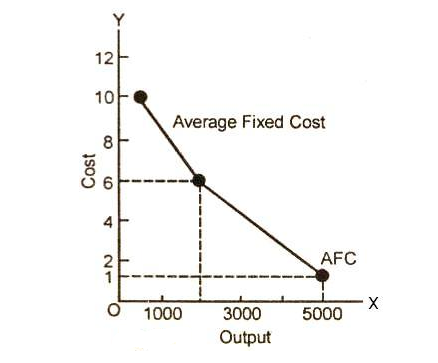# Average Fixed Cost

## Average Fixed Cost Definition

The average fixed cost (AFC) is the fixed cost that does not change with the change in the number of goods and services produced by a company. To put it in a nutshell, the average fixed cost (AFC) is the fixed cost per unit and is calculated by dividing the total fixed cost by the output level.

Since no cost is fixed for a long time, the average fixed cost is only for a short run. When the units of production increase, the average fixed cost per unit decreases. Similarly, when the business produces less units, the average cost increases per unit.

However, only one unit, mostly capital, is fixed. Examples of average fixed cost are the salaries of permanent employees, the mortgage payment on machinery and plant, rent, and more.

## Average Fixed Cost Formula and Example

AFC = Total fixed cost/Output (Q)

If the fixed cost of a pen factory is ₹5,000/- and it produces 500 pens, then the average fixed price will be ₹10/- per unit. Similarly, if the factory produces 1,000 pens, then the cost of a unit will be ₹5/-, and if the total production is 5,000 pens, then the price will come down to ₹1/- per unit.

So, the given example explains that no matter what the output of the product is, the cost remains the same, i.e., ₹5,000/-, whether the production is 500 or 5,000.

### Average Fixed Cost (AFC) in a diagram:In the given example, the cost of the product starts to fall with the increase in production. The price of a pen started at the price of ₹10/- and decreased to ₹1/-. The average fixed cost decreases with the rise in the output. However, the capital ₹5,000/- remains fixed.

Also Read: Important Questions for Production and Costs

This concludes the article on the topic of average fixed cost. It is an important topic of economics for the commerce students. For more such interesting articles, stay tuned to our website.

 Important Topics in Accountancy: